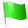# From time precision to Newton's law of gravitation

Date:
Jeudi, 9 Mars, 2017 - 11:30
Lieu:
Bât. 121, salle 123
Nom de l'intervenant:
M. Morfouli (SYRTE, Paris)

According to Westfall “The very heart of the new natural philosophy was mechanics, the science of motion. Mechanics required the measurement of a third dimension, time. The creation of the new world of precision was intimately connected to the success of science in learning to measure time.”

The study of local motion and the free fall in particular, was in the heart of the 17th century research on Natural Philosophy. The establishment of mathematical relations between time, distance, velocity and acceleration was a great challenge, a task carried out by Galileo with fruitful results used later by scholars, including Newton.

In his major work Philosophiae Naturalis Principia Mathematica (1687) Newton presents his theory of universal gravitation. The title of this book reveals an important aim of its author: to introduce mathematical certainty in Physical studies.

In the 3rd book called De Mundi Systemate Newton demonstrates his theory. He compares two free falls: that of a heavy body near the Earth’s surface and that of the Moon towards the Earth’s center, considering only the force of gravity, the so-called Moon test (Prop. IV, Theorem IV). A little further in the same book (Prop. XIX & XX) Newton proposes the application of universal gravitation in order to solve several astronomical and physical problems, such as determining the Earth’s shape. If the theory is correct (the inversed square law) then the force of gravity must be different to different latitudes of the Earth hence the shape of the Earth is not a sphere but an oblate spheroid. One way to confirm this hypothesis is to compare the different lengths of Seconds Pendulums (T=2sec) in different latitudes.

In order to realise these mathematical demonstrations Newton makes use of a number of data, either from observations, essentially astronomical, or from results of calculus. Among the data used, certain concerns time measurement. In the case of the Moon Test one needs to be able to measure or calculate the time of the fall for a certain distance. In the case of the Shape of the Earth one needs to be able to determine the length of a time- measuring instrument. Time measurement thus is well implicated in this affair.

This presentation aims at giving a response to the following question: Is the emerging, increasing precision in time measurement a crucial element for the creation and the confirmation of Newton’s Universal Gravity?

Difficulté: